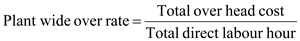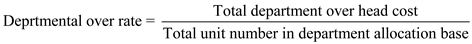## Quiz 7 : Activity-Based Costing and ManagementLooking for Accounting Homework Help?# Quiz 7 : Activity-Based Costing and Management

The two stage process associated with plant wide over rate is as follows: Stage1. In first stage, plant wide overhead rate depends on the direct labour hours. To find the overhead rate the total overhead cost is divided by total labour hours. In which we calculate the single plant wide overhead rate on cost allocation. After calculation, we get plant-wide overhead rate per direct labour.Stage2. In second stage, we calculate the total cost and also calculate per unit cost of each product.

Batch-level driver: A batch-level driver is the driver that is used to allocate the costs using the number of batches of product produced. The batch-level driver varies with the number of batches produced. The batch level driver is calculated by dividing the total overheads with the number of batches produced. Hence, the batch-level driver is consumed by a product each and every unit that batch of products is produced. Therefore, option (a) is correct. Explanation for incorrect options: (b) The batch level driver relates to the batch of units, rather than the unit of product. Hence, option (b) is incorrect. (c) The issue of purchase order is assignment of materials rather than the assignment of overhead costs. Hence, option (c) is incorrect. (d) The number of customer complaints relates to the post production activity and the use of drivers has no relevance. Hence, option (d) is incorrect. (e) As one of the given options (Option (a)) is correct, option (e) was incorrect.

The two stage process for departmental over rates is as follows: Stage1. In this stage, departmental overhead rate depends on the direct labour hours (indirect cost). In first situation, it calculates the departmental wise rate in which there are two departments. Stage2. In this stage, it calculates the overhead rate on the departmental wise rate, then it calculates the cost per unit of each product in which every product have relation with two departments.